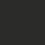# Binomial coefficients

$$\text{Binomial coefficients} { n\choose k}, n,k \in \mathbb{N}_0, k \leq n, \text{are defined as}$$

${n \choose i}=\frac{n!}{i!(n-i)!}$.

$\text{They satisfy}{n \choose i}+{n \choose i-1}={n+1 \choose i} \text{ for } i > 0$

$\text{and also} {n \choose 0}+{n\choose 1}+\cdots+{n \choose n}=2^{n},$

${n \choose 0}-{n\choose 1}+\cdots+(-1)^{n}{n \choose n}=0,$

${n+m \choose k}=\sum\limits_{i=0}^k {n \choose i} {m \choose k-i}.$

$\text{How do I prove that}$

${n+m \choose k}=\sum\limits_{i=0}^k {n \choose i} {m \choose k-i}?$

$\text{(Edit: This is also known as the Vandermonde's Identity.)}$

$\text{Help would be greatly appreciated. (I came across this in a book)}$

$\text{Victor}$

$\text{By the way, I used LaTeX to type the whole note :)}$Note by Victor Loh
6 years, 4 months ago

This discussion board is a place to discuss our Daily Challenges and the math and science related to those challenges. Explanations are more than just a solution — they should explain the steps and thinking strategies that you used to obtain the solution. Comments should further the discussion of math and science.

When posting on Brilliant:

• Use the emojis to react to an explanation, whether you're congratulating a job well done , or just really confused .
• Ask specific questions about the challenge or the steps in somebody's explanation. Well-posed questions can add a lot to the discussion, but posting "I don't understand!" doesn't help anyone.
• Try to contribute something new to the discussion, whether it is an extension, generalization or other idea related to the challenge.

MarkdownAppears as
*italics* or _italics_ italics
**bold** or __bold__ bold
- bulleted- list
• bulleted
• list
1. numbered2. list
1. numbered
2. list
Note: you must add a full line of space before and after lists for them to show up correctly
paragraph 1paragraph 2

paragraph 1

paragraph 2

[example link](https://brilliant.org)example link
> This is a quote
This is a quote
    # I indented these lines
# 4 spaces, and now they show
# up as a code block.

print "hello world"
# I indented these lines
# 4 spaces, and now they show
# up as a code block.

print "hello world"
MathAppears as
Remember to wrap math in $$ ... $$ or $ ... $ to ensure proper formatting.
2 \times 3 $2 \times 3$
2^{34} $2^{34}$
a_{i-1} $a_{i-1}$
\frac{2}{3} $\frac{2}{3}$
\sqrt{2} $\sqrt{2}$
\sum_{i=1}^3 $\sum_{i=1}^3$
\sin \theta $\sin \theta$
\boxed{123} $\boxed{123}$

Sort by:

Deriving Vandermonde's identity is very simple . ( I am going to tell just the procedure ) First what you need to do is just right binomial expansion of $(1+x)^{ n }$ . again rewrite the binomial expansion of$(x+1)^{ m }$ . Note that you should expand $(x+1)^{ m }$ not $(1+x)^{ m }$. now multiplying these two expansions . we get the above summation which is equal to the one of the binomial coefficient in the expansion of $(x+1)^{ m+n }$ Hence prooved

- 6 years, 4 months ago

Here's a combinatorial proof.

Question:

From a group of $m+n$ students consisting of $n$ boys and $m$ girls, how many ways are there to form a team of $k$ students?

${n+m}\choose{k}$.

If that team has $i$ boys, then it'll have $k-i$ girls. How many ways are there to choose $i$ boys from $n$ boys? $n\choose i$.

How many ways are there to choose $k-i$ girls from $m$ girls? $m\choose{k-i}$.

So for a fixed $i$, there are ${n\choose i}{m\choose {k-i}}$ ways to form a team of $k$ students.

Since $i$ could be any number from $0$ to $k$, we add the number ways to form the team for different values of $i$.

So, our final count is,

$\displaystyle \sum_{i=0}^k {n\choose i}{m\choose{k-i}}$

Since answers 1 and 2 are counting the same thing, they must be equal.

[proved]

- 6 years, 4 months ago

yeah, that is the actual proof...by counting in 2 ways.

- 6 years, 4 months ago

Wow

- 6 years, 4 months ago

How do I prove it in a non-combinatorial way?

- 6 years, 4 months ago

Consider the coeff of $x^k$ of both sides in $(1+x)^n (1+x)^m=(1+x)^{n+m}$

- 6 years, 4 months ago

Thank you.

- 6 years, 4 months ago

The last identity is known as Vandermonde's Identity.

- 6 years, 4 months ago

Yay thanks :)

- 6 years, 4 months ago

That's funny that you used $\LaTeX$ on the whole thing. Yes, I will surely work on this cool identity. :D

- 6 years, 4 months ago